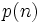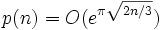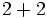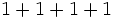Set of unordered integer partitions

Definition

Let$n$ be a nonnegative integer. An unordered integer partition of$n$ is an additive partition of$n$ into positive integers, without any specific ordering on the parts. The set of unordered integer partitions of$n$, sometimes denoted$P(n)$, is the set of all such unordered integer partitions.

The cardinality of this set, also termed the number of unordered integer partitions or partition number, is denoted$p(n)$. We have:$p(n) = O(e^{\pi\sqrt{2n/3}})$.

The set of unordered integer partitions figures in the following ways:

• It is in canonical bijection with the set of conjugacy classes in the symmetric group of degree$n$. The bijection is via the cycle type map, and it is a bijection because cycle type determines conjugacy class.
• It is in canonical bijection with the set of irreducible representations over the rationals (and also, over any algebraic extension of the rationals) of the symmetric group of degree$n$. Further information: Linear representation theory of symmetric groups
• For any fixed prime number$p$, it is in canonical bijection with the set of isomorphism classes of abelian groups of order$p^n$, via the structure theorem for finitely generated abelian groups.

Examples

We have the following small values:$n$$p(n)$ List of partitions Application to conjugacy class structure of symmetric group Application to irreducible representation structure of symmetric group Application to abelian groups of prime power order
0 1 The empty partition trivial group has unique conjugacy class trivial group has unique conjugacy class only the trivial group
1 1 The trivial partition$1$. trivial group has unique conjugacy class trivial group has unique conjugacy class the unique group of prime order, see equivalence of definitions of group of prime order
2 2$2$,$1 + 1$ link link classification of groups of prime-square order
3 3$3$,$2 + 1$,$1 + 1 + 1$ link link link
4 5$4$,$3 + 1$,$2 + 2$,$2 + 1 + 1$,$1 + 1 + 1 + 1$ link link link
5 7$5$,$4 + 1$,$3 + 2$,$3 + 1 + 1$,$2 + 2 + 1$,$2 + 1 + 1 + 1$,$1 + 1 + 1 + 1 + 1$ link link link
9 30 Too long to list
10 42 Too long to list
11 56 Too long to list
12 77 Too long to list
13 101 Too long to list
14 135 Too long to list
15 176 Too long to list
16 231 Too long to list
17 297 Too long to list
18 385 Too long to list
19 490 Too long to list
20 627 Too long to list
21 792 Too long to list
22 1002 Too long to list
23 1255 Too long to list
24 1575 Too long to list